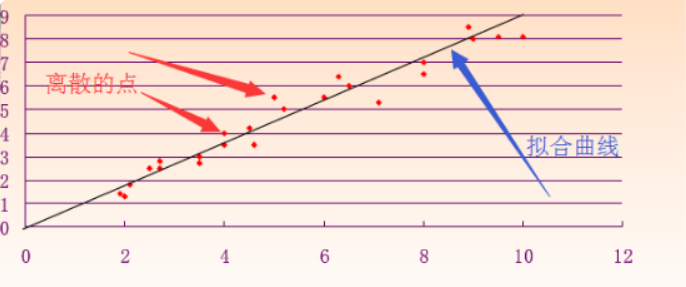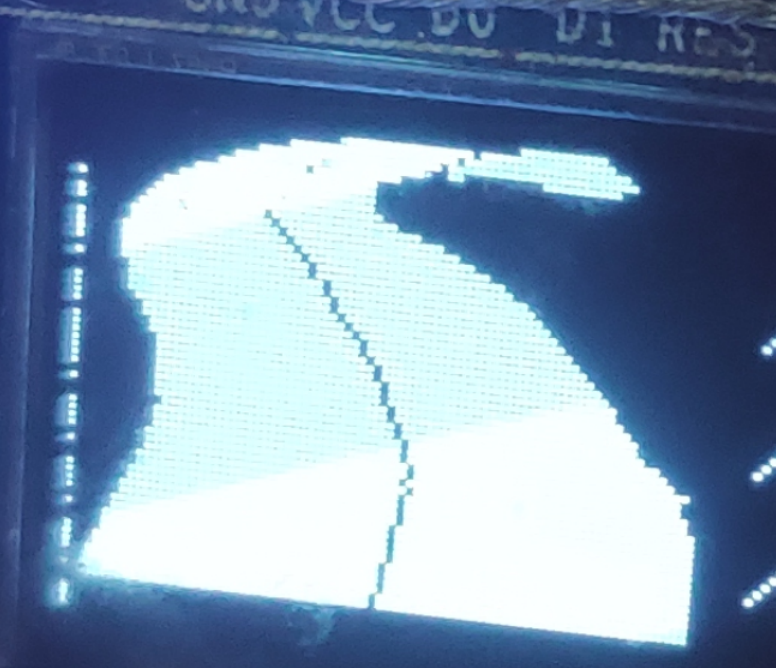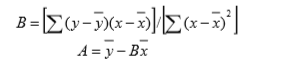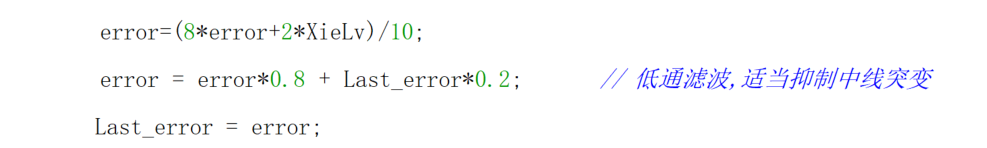最小二乘法：/************************************线性回归计算中线斜率************************************/
// y = Ax+B
int regression(int startline,int endline)
{

int i=0,SumX=0,SumY=0,SumLines = 0;
float SumUp=0,SumDown=0,avrX=0,avrY=0,B,A;
SumLines=endline-startline;   // startline 为开始行， //endline 结束行 //SumLines

for(i=startline;i<endline;i++)
{
SumX+=i;
SumY+=Middle_black[i];    //这里Middle_black为存放中线的数组
}
avrX=SumX/SumLines;     //X的平均值
avrY=SumY/SumLines;     //Y的平均值
SumUp=0;
SumDown=0;
for(i=startline;i<endline;i++)
{
SumUp+=(Middle_black[i]-avrY)*(i-avrX);
SumDown+=(i-avrX)*(i-avrX);
}
if(SumDown==0)
B=0;
else
B=(int)(SumUp/SumDown);
A=(SumY-B*SumX)/SumLines;  //截距
return B;  //返回斜率
}